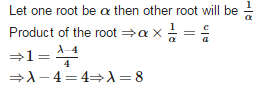Courses

# RD Sharma Test: Quadratic Equations

## 25 Questions MCQ Test Mathematics (Maths) Class 10 | RD Sharma Test: Quadratic Equations

Description
This mock test of RD Sharma Test: Quadratic Equations for Class 10 helps you for every Class 10 entrance exam. This contains 25 Multiple Choice Questions for Class 10 RD Sharma Test: Quadratic Equations (mcq) to study with solutions a complete question bank. The solved questions answers in this RD Sharma Test: Quadratic Equations quiz give you a good mix of easy questions and tough questions. Class 10 students definitely take this RD Sharma Test: Quadratic Equations exercise for a better result in the exam. You can find other RD Sharma Test: Quadratic Equations extra questions, long questions & short questions for Class 10 on EduRev as well by searching above.
QUESTION: 1

### A quadratic equation whose one root is 3 is

Solution:

x = 3 satisfies only the equation x2−5x+6 = 0
As (3)2−5×3+6 = 0
⇒ 9−15+6 = 0
⇒ 15−15 = 0
⇒ 0 = 0
⇒ L.H.S. = R.H.S.

QUESTION: 2

### The common root of 2x2+x−6 = 0 and x2−3x−10 = 0 is

Solution:

Given: p (x) = 2x2 + x - 6 = 0 and q (x) = x2 - 3x — 10 = 0
∴ p (-2) = 2(-2)2 + (-2) - 6 = 0 = 8 - 2 - 6 = 8 - 8 = 0
∴ q (-2) = (-2)2 - 3 (-2) - 10 = 0 = 4 + 6 - 10 = 10 - 10 = 0
Since p (-2) = 0 and q (-2) = 0 therefore, - 2 is the common root of 2x2 + x - 6 = 0 and x2 - 3x - 10 = 0

QUESTION: 3

### In a cricket matchKumble took three wickets less than twice the number of wickets taken by Srinath. The product of the number of wickets taken by these two is 20, then the number of wickets taken by Kumble is

Solution:

Let the number of wickets taken by Srinath be x then the number of wickets taken by Kumble will be 2x−3

According to question, x (2x−3) = 20

⇒ 2x2−3x−20 = 0

⇒ 2x2−8x+5x−20 = 0

⇒ 2x(x−4)+5(x−4) = 0

⇒ (x−4)(2x+5) = 0

⇒ x−4 = 0and 2x+5 = 0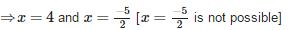Therefore, number of wickets taken by Srinath is 4.
Then number of wickets taken by Kumble = 2 x 4 - 3 = 5

QUESTION: 4

(x2+1)− x2 = 0 has

Solution:

Given: (x2+1)− x= 0
⇒ x+ 1  + 2x − x= 0
⇒ 2x + 1 = 0
⇒ x = −1/2
Therefore, (x2+1)− x2 = 0 has no real roots.

QUESTION: 5

If the sum and product of the roots of the equation kx2+6x+4k = 0 are equal, then k =

Solution: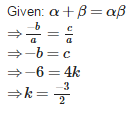QUESTION: 6

One of the roots of the quadratic equation a2x2−3abx+2b2 = 0 is

Solution:

Explanation here:a2x2−3abx+2b= 0
⇒ a2x2−2abx−abx+2b= 0
⇒ ax(ax−2b)−b(ax−2b) = 0
⇒ (ax−b)(ax−2b) = 0
⇒ x−b = 0and ax−2b = 0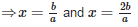QUESTION: 7

The product of two successive integral multiples of 5 is 1050. Then the numbers are

Solution:

Let one multiple of 5 be x then the next consecutive multiple of will be (x+5) According to question,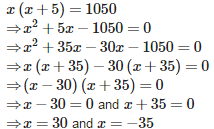x = −35 is not possible therefore x = 30
Then the other multiple of 5 is x+5 = 30+5 = 35
Then the number are 30 and 35.

QUESTION: 8

The angry Arjun carried some arrows for fighting with Bheeshma. With half the arrows, he cut down the arrows thrown by Bheeshma on him and with six other arrows he killed the rath driver of Bheeshma. With one arrow each he knocked down respectively the rath, flag and bow of Bheeshma. Finally with one more than four times the square root of arrows he laid Bheeshma unconscious on an arrow bed. The total number of arrows that Arjun had is

Solution: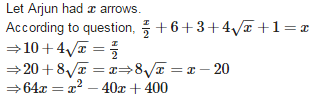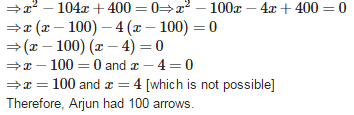QUESTION: 9

Which of the following has no real root?

Solution: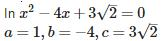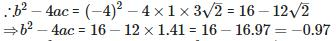Since b2 - 4ac < 0 therefore, x2 - 4x + 3√2 = 0 has no real roots

QUESTION: 10

If ‘sin α’ and ‘cos α’ are the roots of the equation ax2+bx+c = 0 a then b2 =

Solution: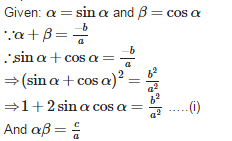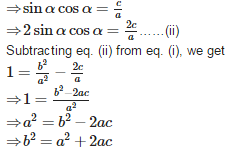QUESTION: 11

The quadratic equation whose roots are 7+√3 and 7−√3 is

Solution: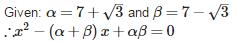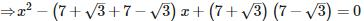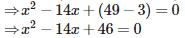QUESTION: 12

The roots of the quadratic equation 9a2b2x2−16abcdx−25c2d2 = 0 are

Solution: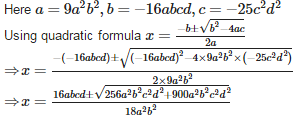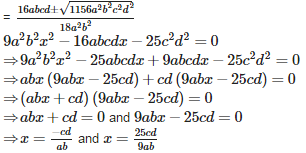QUESTION: 13

The constant that must be added and subtracted to solve the quadratic equation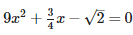by the method of completing the square is

Solution: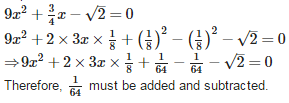QUESTION: 14

Which of the following has two distinct roots?

Solution:

In equation x2+x−5 = 0
a = 1,b = 1,c = −5
∴ b2−4ac = (1)2−4×1×(−5) = 1 + 20 = 21
Since b2−4ac > 0 therefore, x2+x−5 = 0 has two distict roots.

QUESTION: 15

If ‘a’ and ‘b’ are the roots of the equation x2+ax+b = 0, then a+b =

Solution:

Since sum of the roots = -b/a ∴ a+ b = -a/1 = -a

QUESTION: 16

If x = 2 is a root of the quadratic equation 3x2 – px – 2 = 0, then the value of ‘p’ is

Solution:

Given: p(x) = 3x2−px−2 = 0
∴ p(2) = 3(2)2−p(2)−2 = 0
⇒ 12−2p−2 = 0
⇒ −2p = −10
⇒ p = 5

QUESTION: 17

The sum of reciprocals of Sharma’s age 3 years ago and 5 years from now is 1/3, then his present age is

Solution:

Let Sharma’s present age be x years then his age 3 years ago is (x−3) years and 5 years from now is (x+5) years. According to question,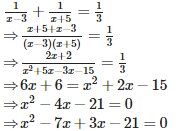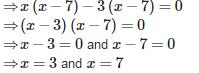But x = 3 does not satisfy the given condition.
Therefore, Sharma's present age is 7 years.

QUESTION: 18

If ax2+bx+c = 0 has equal roots, then c is equal to

Solution:

If ax2+bx+c = 0 has equal roots, then
b2−4ac = 0
⇒ 4ac = b2
⇒ c =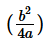QUESTION: 19

If the quadratic equation kx(x – 2) + 6 = 0 has equal roots, then the value of ‘k’ is

Solution: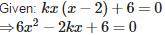If the quadratic equation 6x2 - 2kx + 6 = 0 has equal roots, then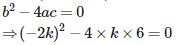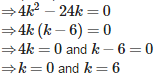QUESTION: 20

If one root of the equation ax2+bx+c = 0 is three times the other, then b2 : ac =

Solution:

Let one root be α then other root will be 3α.
∴ Sum of the roots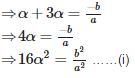And Product of the roots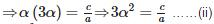Equating the coefficients of α2 and subtracing e.q. (ii) from e.q. (i), we get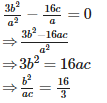QUESTION: 21

5x2+8x+4 = 2x2+4x+6 is a

Solution:

Given: 5x2+8x+4 = 2x2+4x+6
⇒ 5x2−2x2+8x−4x+4−6 = 0
⇒ 3x2+4x−2 = 0
Here, the degree is 2, therefore it is a quadratic equation.

QUESTION: 22

A train travels 360km at a uniform speed. If the speed had been 5 km/hr more, it would have taken 1 hour less for the same journey, then the actual speed of the train is

Solution: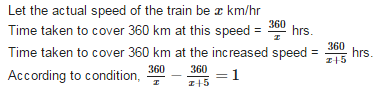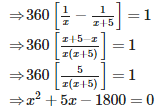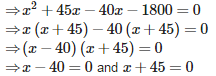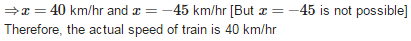QUESTION: 23

Let b = a + c. Then the equation ax2+bx+c = 0 has equal roots if

Solution: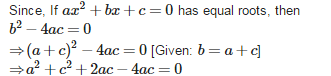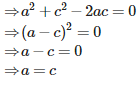QUESTION: 24

The discriminant of the quadratic equation 3√3x2+10x+√3 = 0 is

Solution:

Given: 3√3x2+10x+√3 = 0 Discriminant = b2−4ac = (10)2−4×3√3×√3 = 100−36 = 64

QUESTION: 25

If one root of the equation 4x2−2x+(λ−4) = 0 be the reciprocal of the other, then the value of is

Solution: